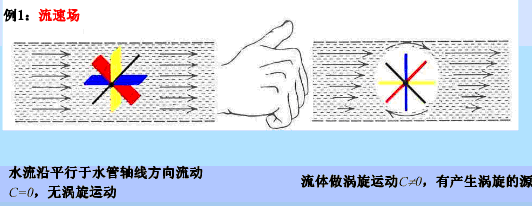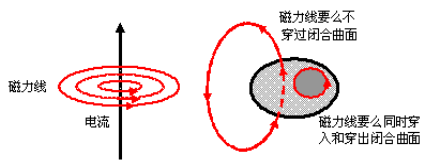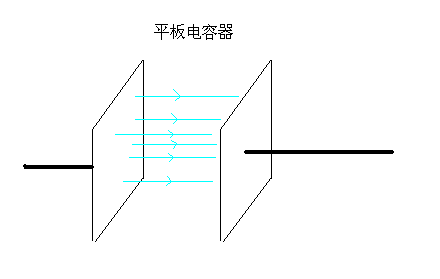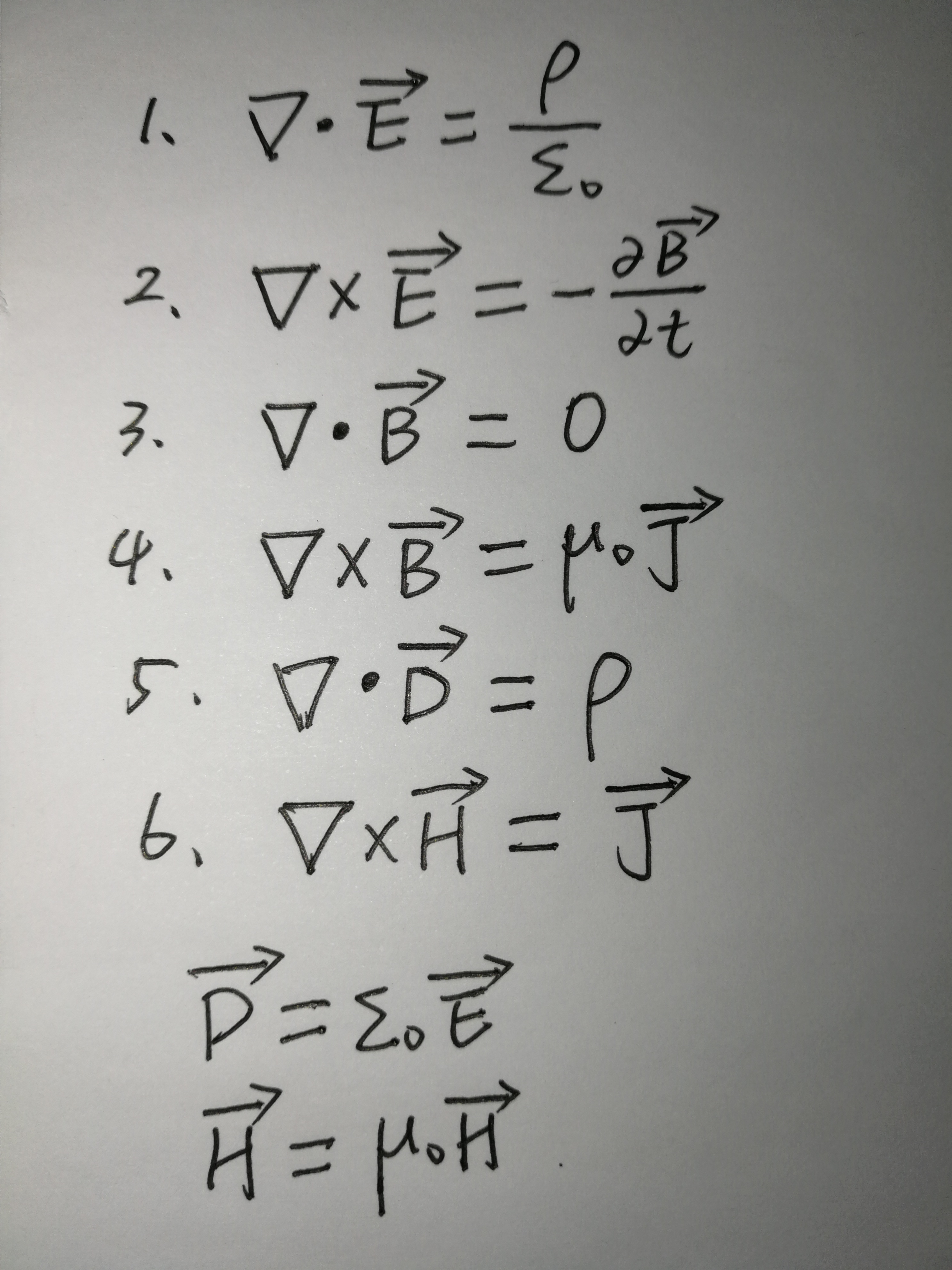sympy有个vector 模块，里面提供了求解标量场、向量场的梯度、散度、旋度等计算,官方参考连接：

http://docs.sympy.org/latest/modules/vector/index.html

sympy中计算梯度、散度和旋度主要有两种方式：
一个是使用∇$\mathrm{\nabla }$$\nabla$算子，sympy提供了类Del()，该类的方法有：cross、dot和gradient，cross就是叉乘，计算旋度的，dot是点乘，用于计算散度，gradient自然就是计算梯度的。

使用sympy计算梯度、散度和旋度之前，首先要确定坐标系，sympy.vector模块里提供了构建坐标系的类，常见的是笛卡尔坐标系， CoordSys3D，根据下面的例子可以了解到相应应用。

（1）计算梯度

## 1 gradient

C = CoordSys3D('C')
delop = Del() # nabla算子

# 标量场 f = x**2*y-xy
f = C.x**2*C.y - C.x*C.y

res = delop.gradient(f, doit=True) # 使用nabla算子
# res = delop(f).doit()

print(res) # (2*C.x*C.y - C.y)*C.i + (C.x**2 - C.x)*C.j

（2）计算散度

## divergence

C = CoordSys3D('C')
delop = Del() # nabla算子

# 向量场 f = x**2*y*i-xy*j
f = C.x**2*C.y*C.i - C.x*C.y*C.j

res = delop.dot(f, doit=True)

# res = divergence(f)

print(res)  # 2*C.x*C.y - C.x，即2xy-x，向量场的散度是标量

（3）计算旋度

## curl

C = CoordSys3D('C')
delop = Del() # nabla算子

# 向量场 f = x**2*y*i-xy*j
f = C.x**2*C.y*C.i - C.x*C.y*C.j

res = delop.cross(f, doit=True)

# res = curl(f)

print(res)  # (-C.x**2 - C.y)*C.k，即(-x**2-y)*k，向量场的旋度是向量
展开全文• ## 梯度、散度和旋度大揭秘

万次阅读 多人点赞 2018-11-06 00:20:36
2、梯度：是一个向量（矢量），表示某一函数在该点处的方向导数沿着该方向取得最大值。 3、通量：在流体运动中，单位时间内流经某单位面积的某属性量，是表示某属性量输送强度的物理量。 4、环量：一个矢量沿一条...
一、基本概念理解
1、方向导数：在函数定义域内的点，对某一方向求导得到的导数。
2、梯度：是一个向量（矢量），表示某一函数在该点处的方向导数沿着该方向取得最大值。
3、通量：在流体运动中，单位时间内流经某单位面积的某属性量，是表示某属性量输送强度的物理量。
4、环量：一个矢量沿一条封闭曲线积分。譬如在流场中任取一条封闭曲线，速度沿该封闭曲线的线积分称为该封闭曲线的速度环量。就像力做功的计算方法一样，形象地称速度环量为速度绕封闭曲线的速度功。
5、散度（divergence）可用于表征空间各点矢量场发散的强弱程度，物理上，散度的意义是场的有源性。当div F>0 ，表示该点有散发通量的正源（发散源）；当div F<0 表示该点有吸收通量的负源（洞或汇）；当div F=0，表示该点无源。
6、旋度是向量分析中的一个向量算子，可以表示三维向量场对某一点附近的微元造成的旋转程度。 这个向量提供了向量场在这一点的旋转性质。旋度向量的方向表示向量场在这一点附近旋转度最大的环量的旋转轴，它和向量旋转的方向满足右手定则。旋度向量的大小则是绕着这个旋转轴旋转的环量与旋转路径围成的面元的面积之比。
7、对散度的理解
散度不为零说明场是有源场，电场有源无旋，磁场无源有旋。电场是由于分离电荷的存在而产生的，所以有源；但磁场目前还没有发现磁单极，所以无源。
8、对旋度的理解
俗话说有图有真相，我们看图说话！我的理解是会“旋转”，是因为受力不均匀所导致。如第一张图水流的例子，水流在垂直的上下平面上大小是相同的，所以左边不产生涡旋。右边里面会产生涡旋，是因为受力不均匀，在垂直的上下这个平面上，越往下，力越小，所以产生了涡旋。
二、梯度、散度和旋度的本质和联系
1、作用对象、运算对象和结果
梯度
作用对象：标量场
运算对象：标量
运算结果：向量（矢量）
散度
作用对象：向量场
运算对象：向量
运算结果：标量
旋度
作用对象：向量场
运算对象：向量
运算结果：向量
1.梯度针对一个标量场（势场），衡量一个标量场的变化方向。梯度为0说明该势场是个等势场。其结果为向量。
2.散度针对一个向量场，衡量一个向量场的单位体积内的场强。散度为0说明这个场没有源头。其结果为标量。
3.旋度针对一个向量场，衡量一个向量场的自旋。旋度为0说明这个场是个保守场（无旋场），保守场一定是某个标量场的梯度场。其结果为矢量。
2、图解 任何标量场的梯度的旋度为0
如下图，电容器内部的电场，其实也就是梯度。对每个垂直方向的平面来说，电势位相等。所以，这就好比刚才水流的那个例子，上下来说受力相等，所以旋度为零。3、电磁场中散度与旋度的求解
在电磁场中任一点处
（1）（▽·E）电场强度的散度==该点处自由电荷的体密度与介电常数之比。
（2）（▽xE）电场强度的旋度== 该点处磁感应强度变化率的负值。
（3）（▽·B）磁感应强度的散度 == 处处等于零。
（4）（▽xB）磁感应强度的旋度 == 该点处电流密度与磁导率的乘积。
（5）（▽·D）电位移的散度== 该点处自由电荷的体密度 .
（6）（▽xH）磁场强度的旋度 == 该点处传导电流密度与位移电流密度 的矢量和。注释：
E 是电场强度矢量
B 是磁感应强度矢量
D 是电位移矢量（也叫电感应强度） 应该还有一个电传导向量 E=D+?
H 是磁场强度矢量    H=B+?
其中内在的联系是：
D=εE
B=μH
大写字母都是矢量
4、场的分类
向量场A，数量场u
▽称为汉密尔顿算子——  ▽·▽=▽2=△
△称为拉普拉斯算子。
1.梯度的旋度▽×▽u=0
梯度场的旋度为0，故梯度场是保守场（无旋场、有势场）。例如重力场。
2.旋度的散度▽·(▽×A)=0
旋度场的散度为0，故旋度场是无源场。例如磁场，磁场本身是其他场的旋度场。
特别说明一下，匀强场是保守场，磁场本身是有旋度的。因此绝对的匀强磁场是不可能的。
拉普拉斯算子△就是偏偏x，偏偏y，偏偏z；拉普拉斯算子是n维欧几里德空间中的一个二阶微分算子，定义为散度。
托克斯公式斯托克斯公式是格林公式的推广。格林公式表达了平面闭区域上的二重积分与其边界曲线上的曲线积分之间的关系。斯托克斯公式则把曲面上的曲面积分与沿着的边界曲线的曲线积分联系起来。


展开全文• 一、散度 矢量 A ⃗ \vec A A 在体积元 d V dV d V 表面上的通量为： (10) d Φ = − A 1 h 2 h 3 d q 2 d q 3 + ( A 1 + ∂ A 1 ∂ q 1 d q 1 ) ( h 2 + ∂ h 2 ∂ q 1 d q 1 ) ( h 3 + ∂ h 3 ∂ q 1 d q 1 ) ...
现有任意一个三维矢量$\vec A$（高维矢量的情况类似）：
$\vec A=A_1\hat e_{q_1}+A_2\hat e_{q_2}+A_3\hat e_{q_3}\tag 1$
$\hat e_{q_i}(i=1,2,3)$为空间中的坐标单位矢量，满足：
\hat e_{q_i}\cdot\hat e_{q_j}=\left\{ \begin{aligned} 0 &, & i\ne j \\ 1 &, & i=j \end{aligned} \right.\tag 2
$\hat e_{q_1}\times\hat e_{q_2}=\hat e_{q_3}\\\hat e_{q_3}\times\hat e_{q_1}=\hat e_{q_2}\\\hat e_{q_2}\times\hat e_{q_3}=\hat e_{q_1}\tag 3$
在此坐标系下，位矢全微分：
$d\vec r=\sum^3_{i=1}h_idq_i\hat e_{q_i}\tag 4$
另有，无限小距离平方为：
$(ds)^2=(d\vec r)^2=\sum^3_{i=1}(h_idq_i)^2\tag 5$
$h_i$为每一坐标方向上的距离微元与该坐标微元的比值，称为该坐标体系在此坐标上的度量系数：
$h_i=\frac{d\vec r\cdot\hat e_{q_i}}{dq_i}\tag 6$
于是，在该坐标体系中，每个坐标方向上的距离微元为：
$dl_i=h_idq_i\tag 7$
面积微元为：
$dS_i=h_jh_kdq_jdq_k，i，j，k互不相等\tag 8$
体积微元为：
$dV=h_1h_2h_3dq_1dq_2dq_3 \tag 9$
一、散度
矢量$\vec A$在体积元$dV$表面上的通量为：
\begin{aligned} d\Phi &=-A_1h_2h_3dq_2dq_3+(A_1+\frac{\partial A_1}{\partial q_1}dq_1)(h_2+\frac{\partial h_2}{\partial q_1}dq_1)(h_3+\frac{\partial h_3}{\partial q_1}dq_1)dq_2dq_3\\ &-A_2h_1h_3dq_1dq_3+(A_2+\frac{\partial A_2}{\partial q_2}dq_2)(h_1+\frac{\partial h_1}{\partial q_2}dq_2)(h_3+\frac{\partial h_3}{\partial q_2}dq_2)dq_1dq_3\\ &-A_3h_1h_2dq_1dq_2+(A_3+\frac{\partial A_3}{\partial q_3}dq_3)(h_1+\frac{\partial h_1}{\partial q_3}dq_3)(h_2+\frac{\partial h_2}{\partial q_3}dq_3)dq_1dq_2\\ &=A_1h_2\frac{\partial h_3}{\partial q_1}dq_1dq_2dq_3+A_1h_3\frac{\partial h_2}{\partial q_1}dq_1dq_2dq_3+\frac{\partial A_1}{\partial q_1}h_2h_3dq_1dq_2dq_3\\ &+A_2h_1\frac{\partial h_3}{\partial q_2}dq_1dq_2dq_3+A_2h_3\frac{\partial h_1}{\partial q_2}dq_1dq_2dq_3+\frac{\partial A_2}{\partial q_2}h_1h_3dq_1dq_2dq_3\\ &+A_3h_1\frac{\partial h_2}{\partial q_3}dq_1dq_2dq_3+A_3h_2\frac{\partial h_1}{\partial q_3}dq_1dq_2dq_3+\frac{\partial A_3}{\partial q_3}h_1h_2dq_1dq_2dq_3\\ &=[\frac{\partial (A_1h_2h_3)}{\partial q_1}+\frac{\partial (A_2h_3h_1)}{\partial q_2}+\frac{\partial (A_3h_1h_2)}{\partial q_3}]dq_1dq_2dq_3 \end{aligned}\tag {10}
（以上忽略微元幂次在4次及以上的高阶项）
$\nabla \cdot \vec A=\frac{d\Phi}{dV} =\frac{1}{h_1h_2h_3}[\frac{\partial (A_1h_2h_3)}{\partial q_1}+\frac{\partial (A_2h_3h_1)}{\partial q_2}+\frac{\partial (A_3h_1h_2)}{\partial q_3}]\tag {11}$
二、旋度
矢量$\vec A$在各个坐标平面上微环的环流为：
\begin{aligned} d\Gamma_1 &=A_2h_2dq_2+(A_3+\frac{\partial A_3}{\partial q_2}dq_2)(h_3+\frac{\partial h_3}{\partial q_2}dq_2)dq_3-(A_2+\frac{\partial A_2}{\partial q_3}dq_3)(h_2+\frac{\partial h_2}{\partial q_3}dq_3)dq_2-A_3h_3dq_3\\ &=A_3\frac{\partial h_3}{\partial q_2}dq_2dq_3+h_3\frac{\partial A_3}{\partial q_2}dq_2dq_3-A_2\frac{\partial h_2}{\partial q_3}dq_2dq_3-h_2\frac{\partial A_2}{\partial q_3}dq_2dq_3\\ &=[\frac{\partial (A_3h_3)}{\partial q_2}-\frac{\partial (A_2h_2)}{\partial q_3}]dq_2dq_3 \end{aligned}\tag {12}
同理可得：
$d\Gamma_2=[\frac{\partial (A_1h_1)}{\partial q_3}-\frac{\partial (A_3h_3)}{\partial q_1}]dq_1dq_3\tag {13}$
$d\Gamma_3=[\frac{\partial (A_2h_2)}{\partial q_1}-\frac{\partial (A_1h_1)}{\partial q_2}]dq_1dq_2\tag {14}$
（以上忽略微元幂次在3次及以上的高阶项）
矢量$\vec A$在各个坐标方向上的环流密度为：
$rot_1\vec A=\frac{d\Gamma_1}{dS_1}=\frac{[\frac{\partial (A_3h_3)}{\partial q_2}-\frac{\partial (A_2h_2)}{\partial q_3}]dq_2dq_3}{h_2h_3dq_2dq_3}=\frac{1}{h_2h_3}[\frac{\partial (A_3h_3)}{\partial q_2}-\frac{\partial (A_2h_2)}{\partial q_3}]\tag {15}$
$rot_2\vec A=\frac{d\Gamma_2}{dS_2}=\frac{[\frac{\partial (A_1h_1)}{\partial q_3}-\frac{\partial (A_3h_3)}{\partial q_1}]dq_1dq_3}{h_1h_3dq_1dq_3}=\frac{1}{h_1h_3}[\frac{\partial (A_1h_1)}{\partial q_3}-\frac{\partial (A_3h_3)}{\partial q_1}]\tag{16}$
$rot_3\vec A=\frac{d\Gamma_3}{dS_3}=\frac{[\frac{\partial (A_2h_2)}{\partial q_1}-\frac{\partial (A_1h_1)}{\partial q_1}]dq_1dq_2}{h_1h_2dq_1dq_2}=\frac{1}{h_1h_2}[\frac{\partial (A_2h_2)}{\partial q_1}-\frac{\partial (A_1h_1)}{\partial q_2}]\tag{17}$
故矢量$\vec A$的旋度为：
\begin{aligned} \nabla\times \vec A &=\hat e_{q_1}rot_1\vec A+\hat e_{q_2}rot_2\vec A+\hat e_{q_3}rot_3\vec A\\ &=\frac{1}{h_2h_3}[\frac{\partial (A_3h_3)}{\partial q_2}-\frac{\partial (A_2h_2)}{\partial q_3}]\hat e_{q_1}+\frac{1}{h_1h_3}[\frac{\partial (A_1h_1)}{\partial q_3}-\frac{\partial (A_3h_3)}{\partial q_1}]\hat e_{q_2}+\frac{1}{h_1h_2}[\frac{\partial (A_2h_2)}{\partial q_1}-\frac{\partial (A_1h_1)}{\partial q_2}]\hat e_{q_3}\\ &=\frac{1}{h_1h_2h_3}\left |\begin{matrix} h_1\hat e_{q_1} & h_2\hat e_{q_2} & h_3\hat e_{q_3} \\\\ \frac{\partial}{\partial q_1} & \frac{\partial}{\partial q_2} & \frac{\partial}{\partial q_3}\\\\ h_1A_1 & h_2A_2 & h_3A_3 \end{matrix} \right | \end{aligned}\tag {18}
三、散度以及旋度的矩阵形式
$\vec A= \begin{bmatrix} A_1\\\\ A_2\\\\ A_3 \end{bmatrix}\tag{19}$
$\nabla \cdot\vec A=\frac{1}{h_1h_2h_3} \begin{bmatrix} \frac{\partial}{\partial q_1}\\\\ \frac{\partial}{\partial q_2}\\\\ \frac{\partial}{\partial q_3} \end{bmatrix}^T \begin{bmatrix} h_2h_3 & 0 & 0\\\\ 0 & h_3h_1 & 0\\\\ 0 & 0 & h_1h_2 \end{bmatrix} \begin{bmatrix} A_1\\\\A_2\\\\A_3 \end{bmatrix}\tag{20}$
$\nabla \times\vec A=\frac{1}{h_1h_2h_3} \begin{bmatrix} h_1 & 0 & 0\\\\ 0 & h_2 & 0\\\\ 0 & 0 & h_3 \end{bmatrix} \begin{bmatrix} 0 & -\frac{\partial}{\partial q_3} & \frac{\partial}{\partial q_2}\\\\ \frac{\partial}{\partial q_3} & 0 & -\frac{\partial}{\partial q_1}\\\\ -\frac{\partial}{\partial q_2} & \frac{\partial}{\partial q_1} & 0 \end{bmatrix} \begin{bmatrix} h_1 & 0 & 0\\\\ 0 & h_2 & 0\\\\ 0 & 0 & h_3 \end{bmatrix} \begin{bmatrix} A_1\\\\A_2\\\\A_3 \end{bmatrix}\tag{21}$
附：算子运算规则（举例说明）：
$\phi(x,y,z)$与$\psi(x,y,z)$是两个函数，算子$\nabla$（$\nabla=(\hat e_x\frac{\partial}{\partial x}+\hat e_y\frac{\partial}{\partial y}+\hat e_z\frac{\partial}{\partial z})$）的运算规则如下：
$\phi(x,y,z)\nabla\psi(x,y,z)=\phi\frac{\partial\psi}{\partial x}\hat e_x+\phi\frac{\partial\psi}{\partial y}\hat e_y+\phi\frac{\partial\psi}{\partial z}\hat e_z\tag{22}$


展开全文矩阵
• 圆柱和球坐标下的散度和旋度：使用向量运算恒等式将散度和旋度转换为梯度运算。见第一行。   梯度的旋度为0； 旋度的散度为0。   梯度总是指向函数增长最快的方向。   旋度的散度为零，意味...
• 该方法不仅再现了向量场散度和旋度参数的几何关联性及其微分特性, 更实现了多维统一与坐标无关的计算. 基于北美风场的模拟实验显示, 该算法比基于梯度的间接求解算法具有更好的对比度与平滑性特征, 且具有较强的抗...
• 的定义       已给向量F⃗=P i⃗+Q j⃗+R k⃗\vec F = P\,\vec i + Q\,\vec j + R\,\vec k F=Pi+Qj​+Rk ...则该向量的旋表示为rot  F⃗=(Ry−Qz)i⃗+(Pz...可视化 线性代数
• 问答回顾：如何直观形象的理解梯度散度FRANK WANG个人微信订阅号：振曰（zhenyue_frank) 振曰（微信：zhenyue_frank）2014年我在知乎里回答了“如何直观形象的理解梯度，散度，旋？”的问题，受到广泛赞同，...基础知识
• 散度的讨论应从向量和向量场说起。向量是数学中研究多维计算的基本概念。比如，速度可以分解为相互独立的分量，则速度就是一个多维的向量。假如空间中的每一个位置都有一个向量属性的话，这个空间就叫做向量场。比如...
• 梯度、散度和旋度的定义及公式表达 ​ 梯度是个向量 或表示为 散度是个标量 设有一个向量场 通量可写为 则散度 并有运算关系式 旋度是个向量 rotA或...
• 电场就是无旋,它是有源.稳恒磁场是有旋无源,其磁场强度不受其他因素影响,而非稳恒磁场里的电生磁中的磁场是由电流产生的,不通过电流时即不产生磁场,这种磁场就是有源有旋,这就是电磁铁的原理..用定义已经...
• ## 梯度、散度、旋度

千次阅读 2012-10-22 22:39:32
梯度、散度和旋度的定义及公式表达 梯度是个向量 或表示为 散度是个标量 设有一个向量场 通量可写为 则散度 并有运算关系式 旋度是个向量 rotA或curlA 或可以写成 例如求F沿路径...
• ∇：向量微分算子、哈密尔顿算子、Nabla算子、劈形算子，倒三角算子是一个微分算子。 Strictly speaking,∇del is not a specific operator, but rather a convenient mathematical notation for those three ...
• 在一个纯量中，梯度的计算结果会是”在每个位置都算出一个向量，而这个向量的方向会是在任何一点上从其周围（极接近的周围，学过微积分该知道甚么叫极限吧?）纯量值最小处指向周围纯量值最大处。而这个向量的大小...梯度
• ## 旋度和散度

千次阅读 多人点赞 2019-04-01 00:33:47
如上图这个向量场，假设向量代表个点的速度，这种流体满足一种非常奇怪的非物理特性，在如下图的点，流体似乎在虚无中向外抛射出流体。其他点则像有流体流入。 散度所告诉你的正式，向外发射或向内吸收的程度，...
• 这是一个可用于计算电磁散度、梯度和旋度的工具。 您可以在此处找到如何使用它的示例： http : //behindthesciences.com/gradient-divergence-and-curl/ 如果您对以后的帖子有任何问题或建议，请给我们发电子...matlab
• 它们的推导过程与梯度、散度、与旋具有密切的关系。本文主要通过对三维空间中梯度、散度与旋的解释来分析麦克斯韦方程组。为了方便表示，这里f(x,y,z)f(x,y,z)f(x,y,z)表示一个含有三个变量x,y,z的一个函数，...梯度 麦克斯韦方程 微分几何
• 在向量微积分中，标量场的梯度是一个向量场。标量场中某一点上的梯度指向标量场增长最快的方向，梯度的长度是这个最大的变化率。更严格的说，从欧氏空间Rn到R的函数的梯度是在Rn某一点最佳的线性近似。在这个意义上...抽象代数
• ## 梯度、散度、旋度的关系

万次阅读 多人点赞 2017-10-14 14:49:57
向量场 数量场 有源场 无源场 保守场（无旋场）有旋场（非保守场）   保守场=有势场=无旋场------环流等于零! 有源场-------闭合曲面的通量不等于零!------这些是指场的宏观特性!     3.含时磁场
•  偏导，方导，梯度，散度，旋是高等数学的几个基本概念，在图形学中会经常用到，这里重新来学习一下，同时也当作一个记录。  偏导 定义：一个多变量的函数的偏导数是它关于其中一个变量的导数，而保持其他变量...算法 数学
• 我见过最清晰的–理解梯度，散度，旋 梯度: 运算的对像是纯量,运算出来的结果会是向量在一个纯量中, 梯度的计算结果会是"在每个位置都算出一个向量,而这个向量的方向会是在任何一点上从其周围(极接近的周围...机器学习
• 首先梯度和旋度是向量，而散度是标量。 1.梯度针对一个数量场（势场），衡量一个数量场的变化方向。梯度为0说明该势场是个等势场。其结果为向量。 2.散度针对一个向量场，衡量一个向量场的单位体积内的场强。...
• 6. 求矢量的旋。 例10(curl)： [x, y, z] = meshgrid(-3:1:3); u=3.*x.^2 + 2.*y.^2 + z.^2; v=x.^2 + 3.*y.^2 + 2.*z.^2; w=2.*x.^2 + 3.*y.^2 + z.^2; [curlx, curly, curlz]=curl(x, y,z, u, ...Matlabmath div